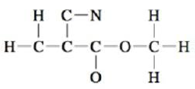# A common trait of simple organic compounds is to have Lewis structures where all atoms have a formal charge of zero. Consider the following incomplete Lewis structure for an organic compound called methyl cyanoacrylate, the main ingredient in Super Glue. Draw a complete Lewis structure for methyl cyanoacrylate in which all atoms have a formal charge of zero.### Chemistry: An Atoms First Approach

2nd Edition
Steven S. Zumdahl + 1 other
Publisher: Cengage Learning
ISBN: 9781305079243

#### Solutions

Chapter
Section### Chemistry: An Atoms First Approach

2nd Edition
Steven S. Zumdahl + 1 other
Publisher: Cengage Learning
ISBN: 9781305079243
Chapter 3, Problem 101E
Textbook Problem
39 views

## A common trait of simple organic compounds is to have Lewis structures where all atoms have a formal charge of zero. Consider the following incomplete Lewis structure for an organic compound called methyl cyanoacrylate, the main ingredient in Super Glue.Draw a complete Lewis structure for methyl cyanoacrylate in which all atoms have a formal charge of zero.

Interpretation Introduction

Interpretation: The complete Lewis structure from the given incomplete structure is to be drawn for the molecule methyl cynoacrylate such that all atoms should have zero formal charge.

Concept introduction: The Lewis structure is also known as dot structure. This structure depicts the bonding between atoms and the lone pairs of electrons if exists.

Formal charge is a charge given to an atom with assumption that electrons are shared equally between atoms in all chemical bonds irrespective of their electronegativity.

To determine: The Lewis structure of methyl cynoacrylate (C5H5NO2) in which all atoms have formal charge zero.

### Explanation of Solution

To determine: The Lewis structure of the molecule methyl cyanoacrylate (C5H5NO2) .

A common trait of simple organic compounds is to have Lewis structures where all atoms have a formal charge of zero.

The given diagram of methyl cyanoacrylate is,

Figure 1

The first step in determining the Lewis structure is to determine the number of valence electrons. The atomic number of carbon (C) is 6 and its electronic configuration is,

1s22s22p2

The valence electron of carbon is 4 .

The atomic number of nitrogen (N) is 7 and its electronic configuration is,

1s22s22p3

The valence electron of nitrogen is 5 .

The atomic number of oxygen (O) is 8 and its electronic configuration is,

1s22s22p4

The valence electron of oxygen is 6 .

The atomic number of hydrogen is 1 and its electronic configuration is,

1s1

The valence electron of hydrogen is 1 .

The molecule C5H5NO2 is made of five hydrogen atoms, one nitrogen atom, two carbon atoms and two oxygen atom; hence,

Total number of valence electrons in C5H5NO2 =(5×4)+(5×1)+5+(2×6)=42

Each hydrogen atom requires one electron to complete the duplet, oxygen atom require two electrons, carbon atom requires four electrons and nitrogen atom require  3 electrons to complete their octet. Hence, the mutual sharing of electrons takes place.

Thus, the Lewis structure of C5H5NO2 is,

### Still sussing out bartleby?

Check out a sample textbook solution.

See a sample solution

#### The Solution to Your Study Problems

Bartleby provides explanations to thousands of textbook problems written by our experts, many with advanced degrees!

Get Started

Find more solutions based on key concepts
The length 500 m can also be expressed as nm.

Introductory Chemistry: A Foundation

The smallest unit of life is the _____. a. atom b. molecule c. cell d. organism

Biology: The Unity and Diversity of Life (MindTap Course List)

Fat breakdown without carbohydrate causes ketones to build up in the tissues and blood and be excreted in the u...

Nutrition: Concepts and Controversies - Standalone book (MindTap Course List)

Consider the voltage phasor in Figure 32.4, shown at three instants of time. (i) Choose the part of the figure,...

Physics for Scientists and Engineers, Technology Update (No access codes included)